## In a series circuit, a generator (1300 Hz, 12.0 V) is connected to a 14.0- resistor, a 4.40-μF capacitor, and a 6.00-mH inductor. Find the v

Question

In a series circuit, a generator (1300 Hz, 12.0 V) is connected to a 14.0- resistor, a 4.40-μF capacitor, and a 6.00-mH inductor. Find the voltages across (a) the resistor, (b) the capacitor, and (c) the inductor. Note: The ac current and voltage are rms values and power is an average value unless indicated otherwise.

in progress 0
5 months 2021-08-19T04:29:44+00:00 1 Answers 1 views 0

(a) 2.8 V

(b) 5.6 V

(c) 9.8 V

Explanation:

Given:

Frequency of the generator (f) = 1300 Hz)

Terminal voltage (V) =12.0 V

Resistance of resistor (R) = 14.0 Ω

Capacitance of capacitor (C) = 4.40 μF = 4.40 × 10⁻⁶ F

Inductance of the inductor (L) = 6.00 mH = 6.00 × 10⁻³ H

In order to find the voltages across each, we first need to find the reactance and impedance.

Reactance of the inductor is given as: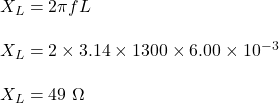Reactance of the capacitor is given as: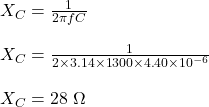Now, impedance is given as: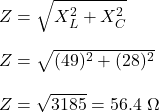Current across the circuit is given as: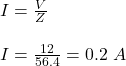As resistor, capacitor and inductor are connected in series, the current across each of them is same and equal to total current in the circuit.

(a)

Voltage across the resistor is given as: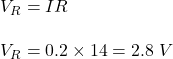Therefore, the voltage across resistor is 2.8 V.

(b)

Voltage across the capacitor is given as: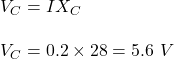Therefore, the voltage across the capacitor is 5.6 V.

(c)

Voltage across the inductor is given as: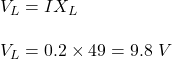Therefore, the voltage across the inductor is 9.8 V.